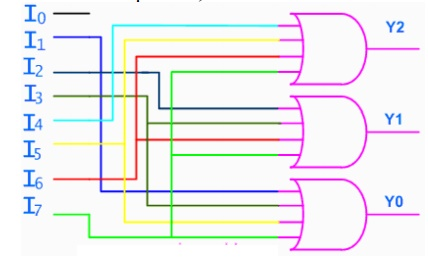Home | | Digital Electronics | Encoders

# Encoders

An encoder is a combinational circuit that performs the inverse operation of a decoder.

ENCODERS

An encoder is a combinational circuit that performs the inverse operation of a decoder. If a device output code has fewer bits than the input code has, the device is usually called an encoder. e.g. 2n-to-n, priority encoders.

The simplest encoder is a 2n-to-n binary encoder, where it has only one of 2n inputs = 1 and the output is the n-bit binary number corresponding to the active input. It can be built from OR gates## Octal-to-Binary Encoder

Octal-to-Binary take 8 inputs and provides 3 outputs, thus doing the opposite of what the 3-to-8 decoder does. At any one time, only one input line has a value of 1. The figure below shows the truth table of an Octal-to-binary encoder.

## Truth TableFor an 8-to-3 binary encoder with inputs I0-I7 the logic expressions of the outputs Y0-Y2 are:

Y0 = I1 + I3 + I5 + I7

Y1= I2 + I3 + I6 + I7

Y2 = I4 + I5 + I6 +I7

Based on the above equations, we can draw the circuit as shown belowStudy Material, Lecturing Notes, Assignment, Reference, Wiki description explanation, brief detail
Digital Electronics : Combinational Circuits : Encoders |